# Trained mapping

We create a mapping based on some reference data referring to a small geographic area we can’t resolve with our data.explanation on capture rate

We don’t know a priori how each cell contributes to the measurement of visitors and we create an iterative process to estabilsh the contribution of each cell.

## Consistency in reference data

Reference data have internal consistency that varies from location to location.

We first control how stable is the correlation adding noise.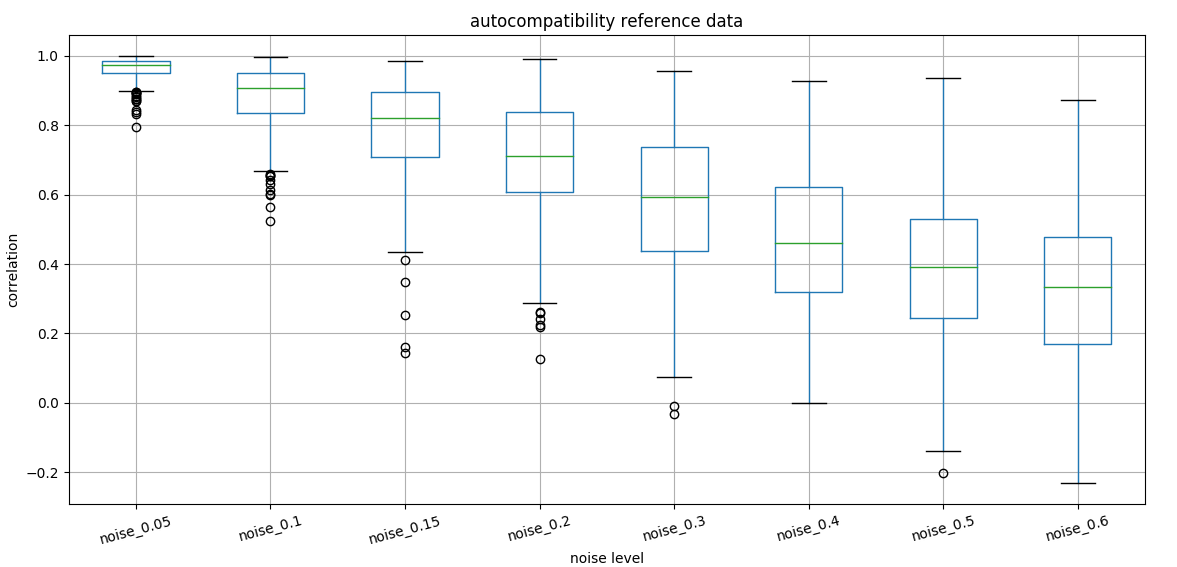stability of correlation introducing noise

We can show as well that noise at the hourly level does not change correlation as fast as for daily values.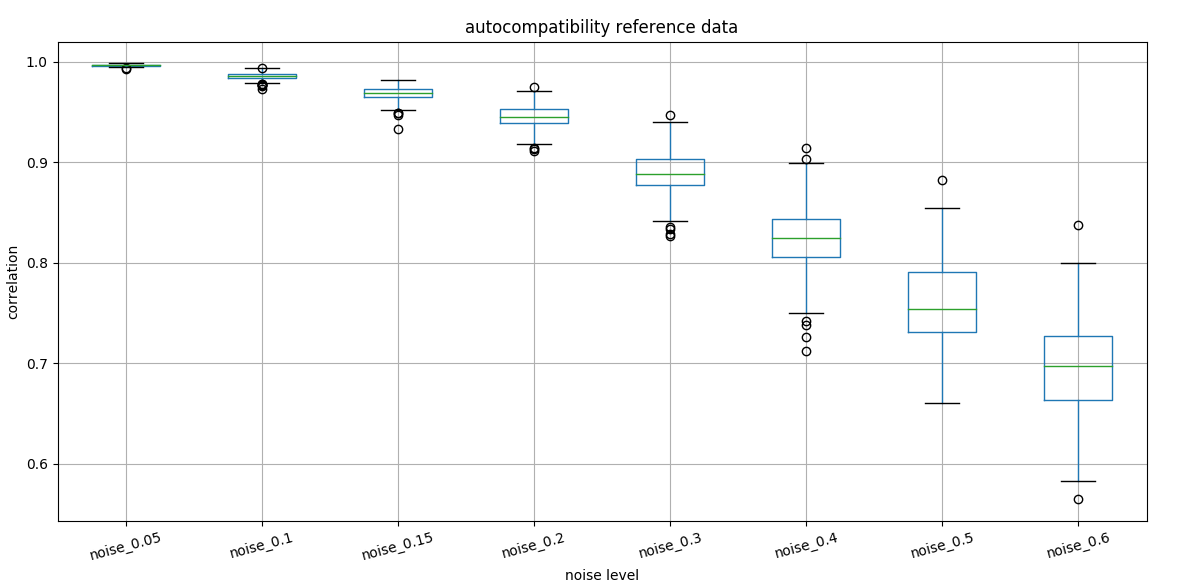noise on reference data

We see the effect of noise on reference data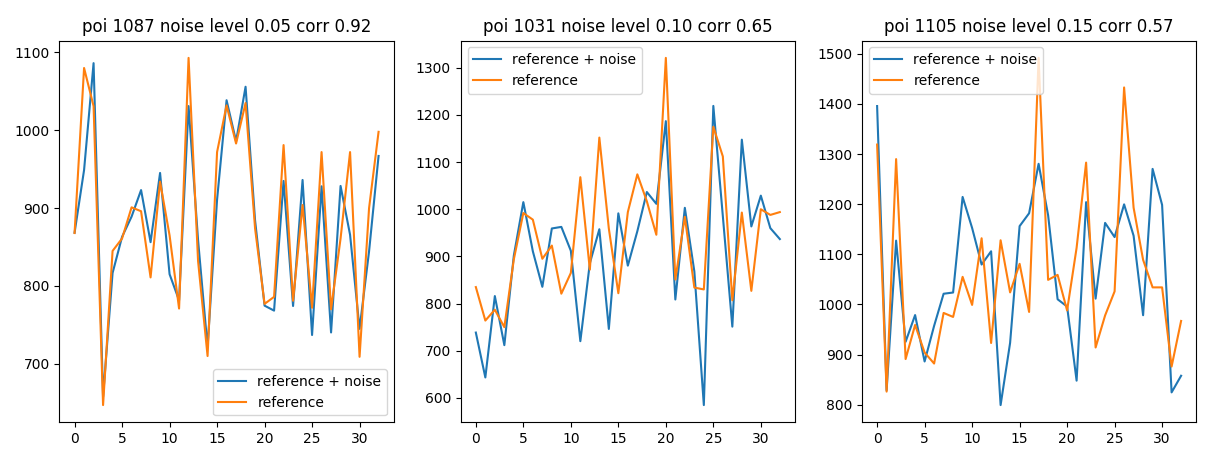noise on reference data

Already with 15% noise we can’t match the reference data with correlation 0.6 at a constant relative error.

If we look at historical data (variance of days with the same isocalendar date) we see that holiday have a big contribution in deviation.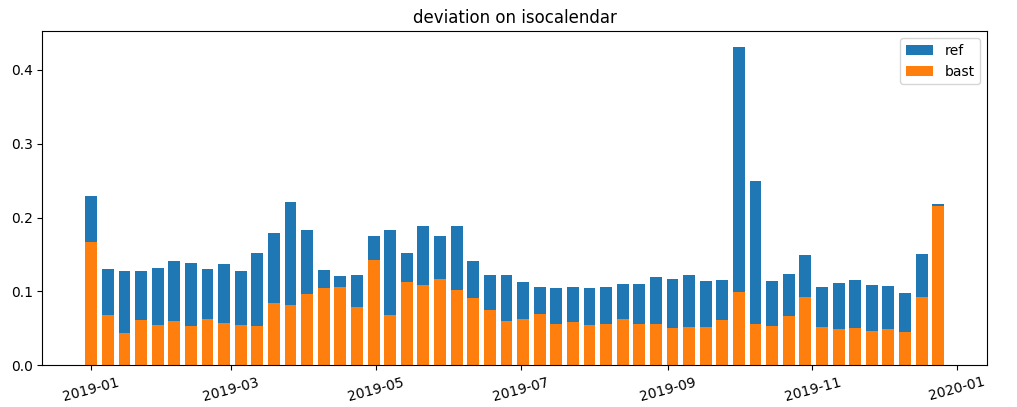deviation on isocalendar

### forecastability

We quantify the forcastability of a customer time series running a long short term memory on reference data.

We can see that some reference data are easy forcastable by the model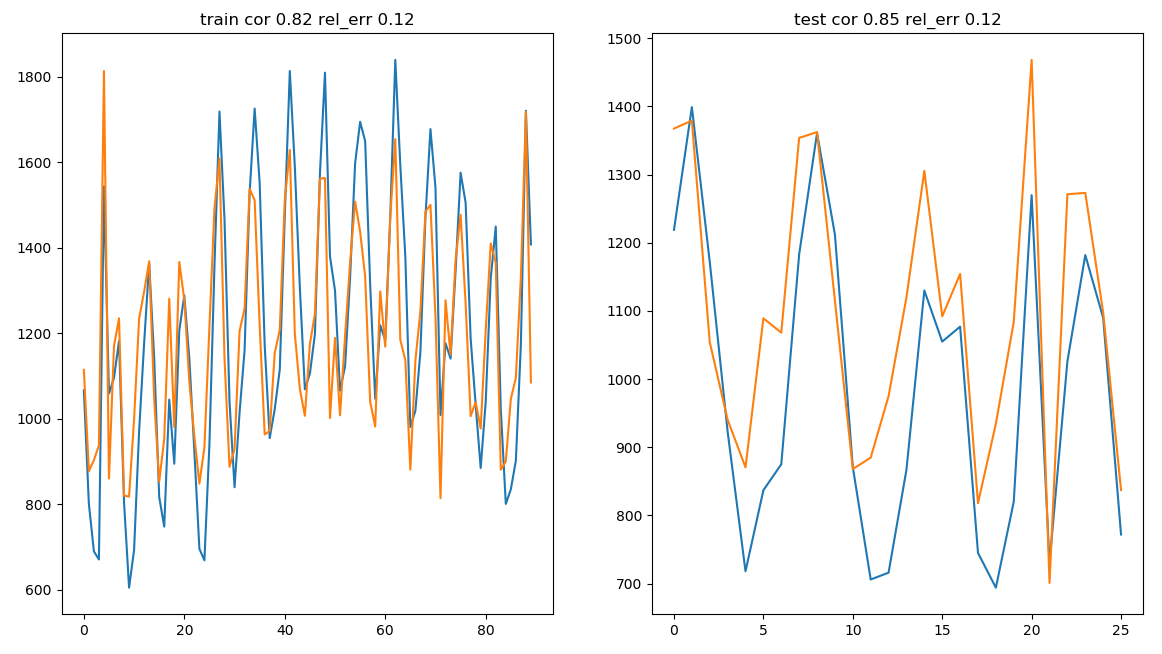example of good forecastable model

While some are not understood from the neural network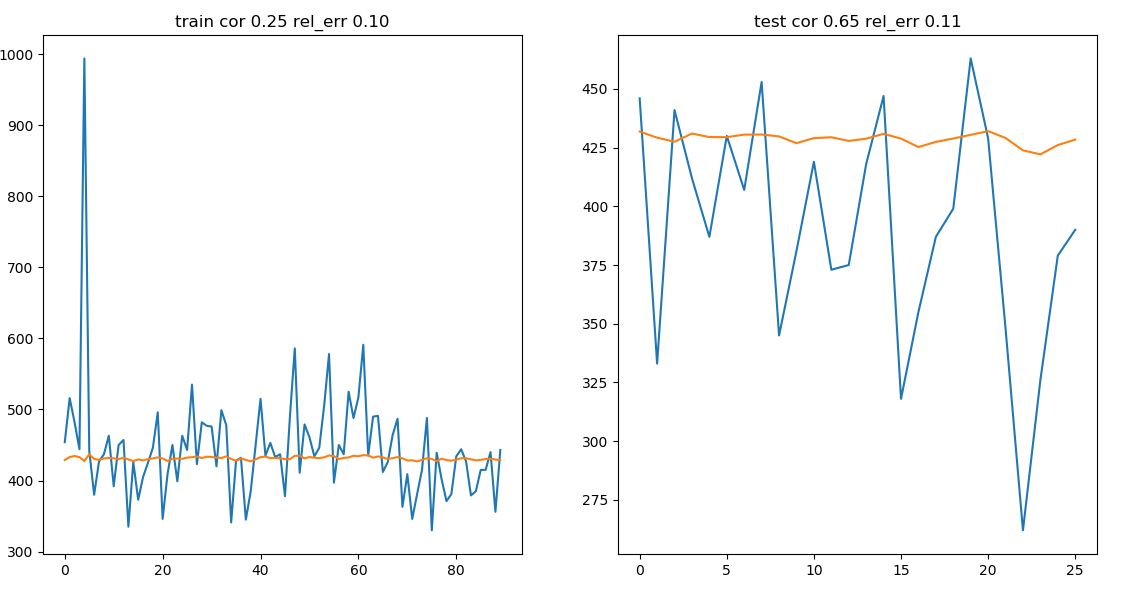example of bad forecastable model

## Data preparation

We use the following job file to produce the activity with the filters:filter on activities

• max 2 hours stay
• 20 km previous trip distance
• chirality (previous direction)

Filtering does not change the overall curves but just the niveau

Filtering options:

name tripEx distance duration chirality
t4 10.5 20km 30min-2h True
t4_10 10.5 10km 15min-2h True
t4_p11 11.6 20km 15min-2h True
t4_p11_d20-notime 11.6 20km 15min-2h True
t4_p11_d10 11.6 10km 15min-2h True
t4_p11_d20 11.6 20km 15min-2h True
t4_p11_d30 11.6 30km 15min-2h True
t4_p11_d40 11.6 40km 15min-2h True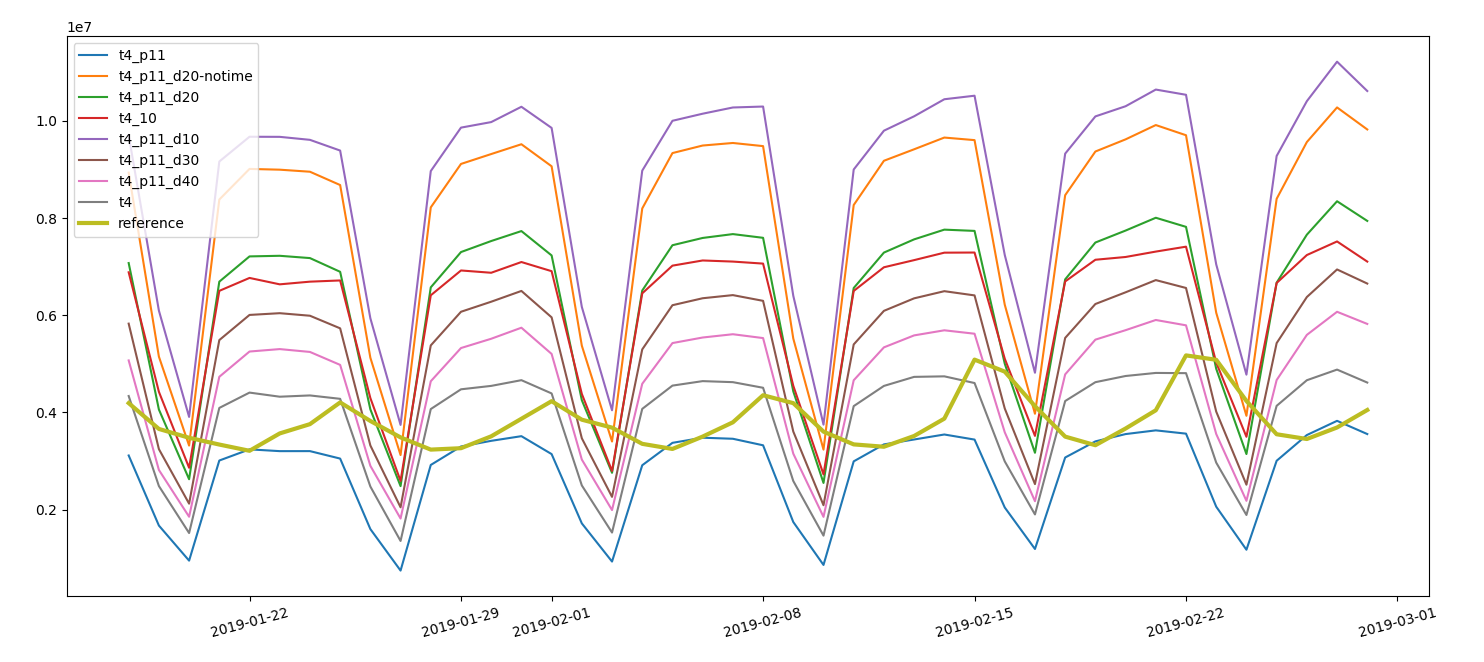effect of filters on curves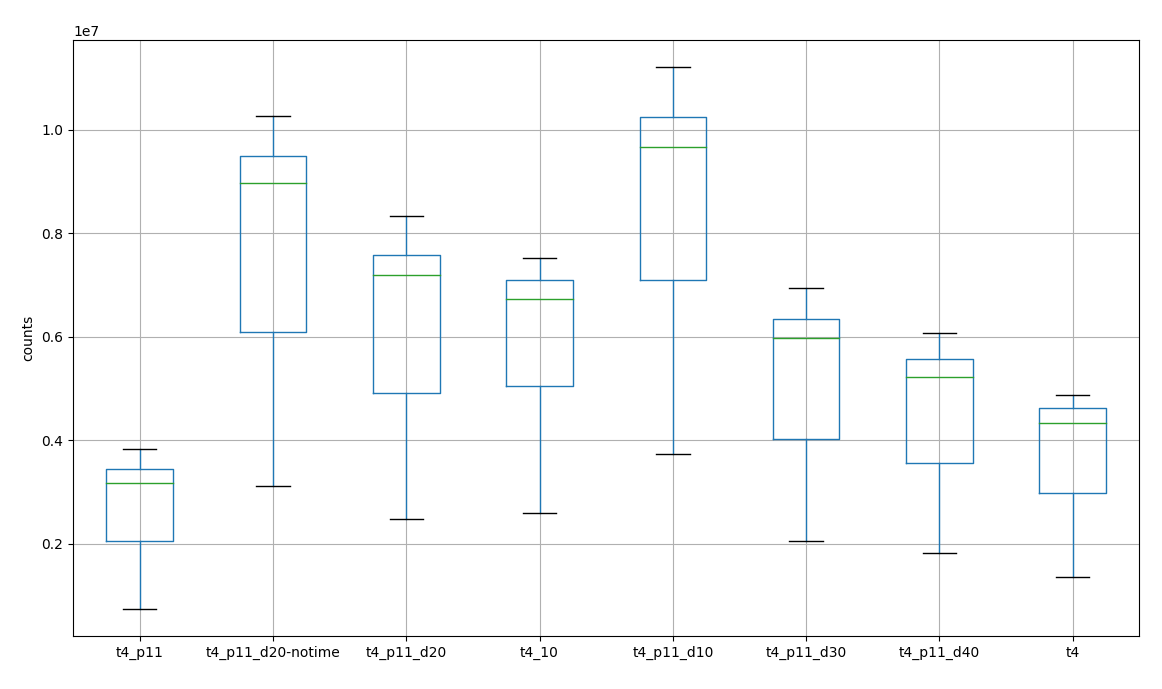counts changed by filtering

We calculate daily values on cilac basis.

The tarball is downloaded and processed with an etl script which uses the a function to unpack the tar and process the output with spark.

## Mapping weighting

We filter the cells using only the first 20 ones whose centroid is close to the poi.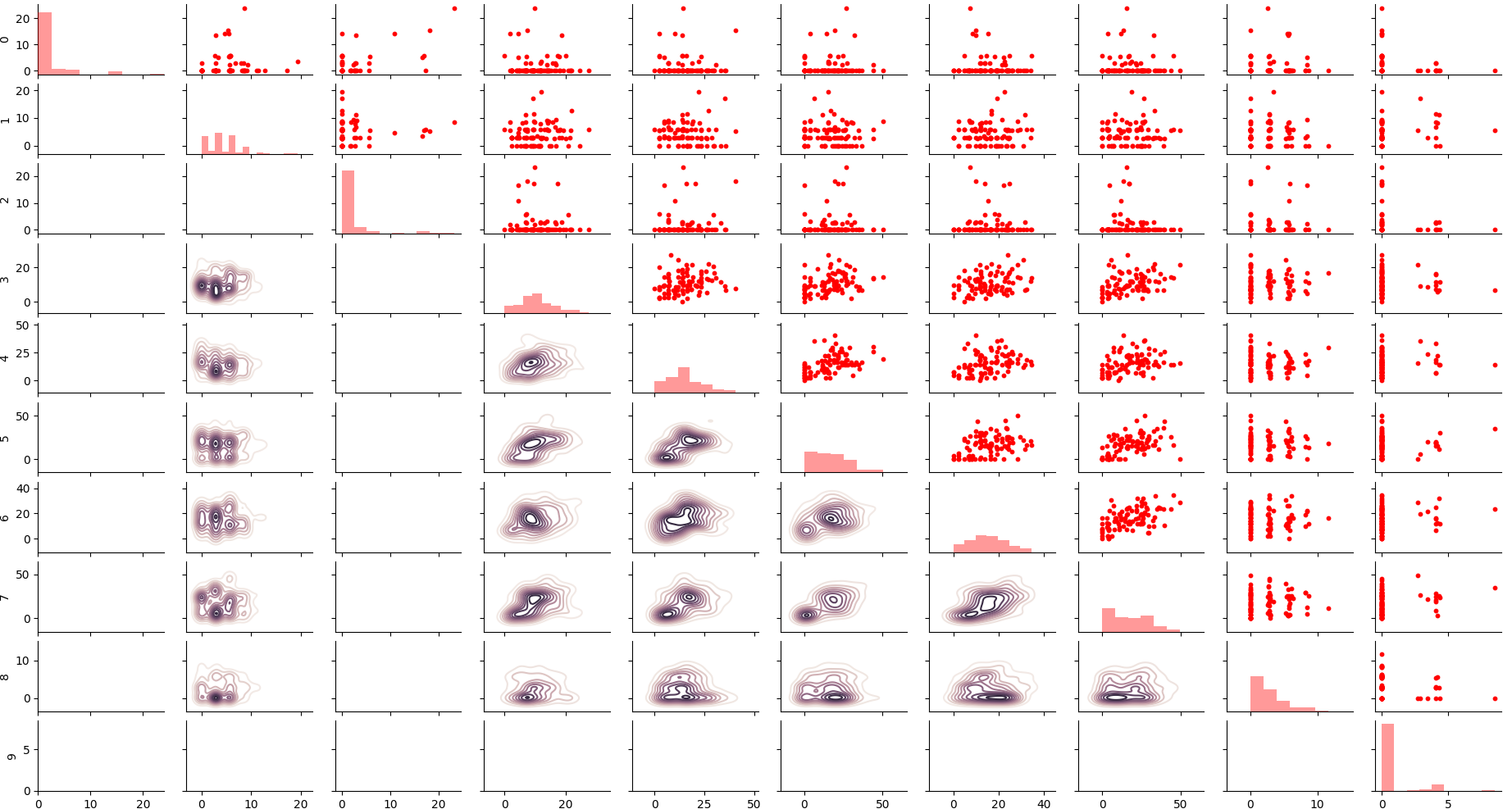some cells are not correlating within each other

At first sight the sum of activities doesn’t mirror the reference data, neither in sum nor in correlation.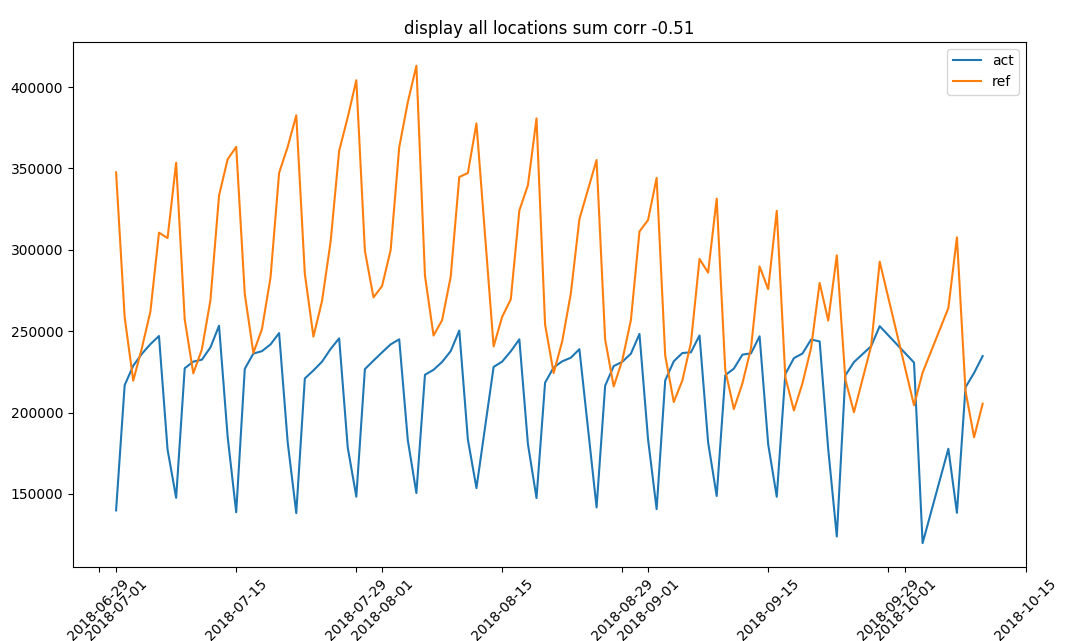sum of overall activities and visits

We use linLeastSq to find the best linear weights for each cell contributing to measure the activities at the location.

   def ser_sin(x,t,param): #weights times activities
return x*t.sum(axis=0)
def ser_fun_min(x,t,y,param): #minimizing total sum
return ser_sin(x,t,param).sum() - y.sum()
x0 = X.sum(axis=0) #starting values
x0 = x0/x0.mean() #normalization
res = least_squares(ser_fun_min,x0,args=(X,y,x0)) #least square optimization
beta_hat = res['x']

function that optimizes cells weights minimizing the total sum difference wrt reference data

We iterate over all locations to find the best weight set using a minimum number of cells (i.e. 5)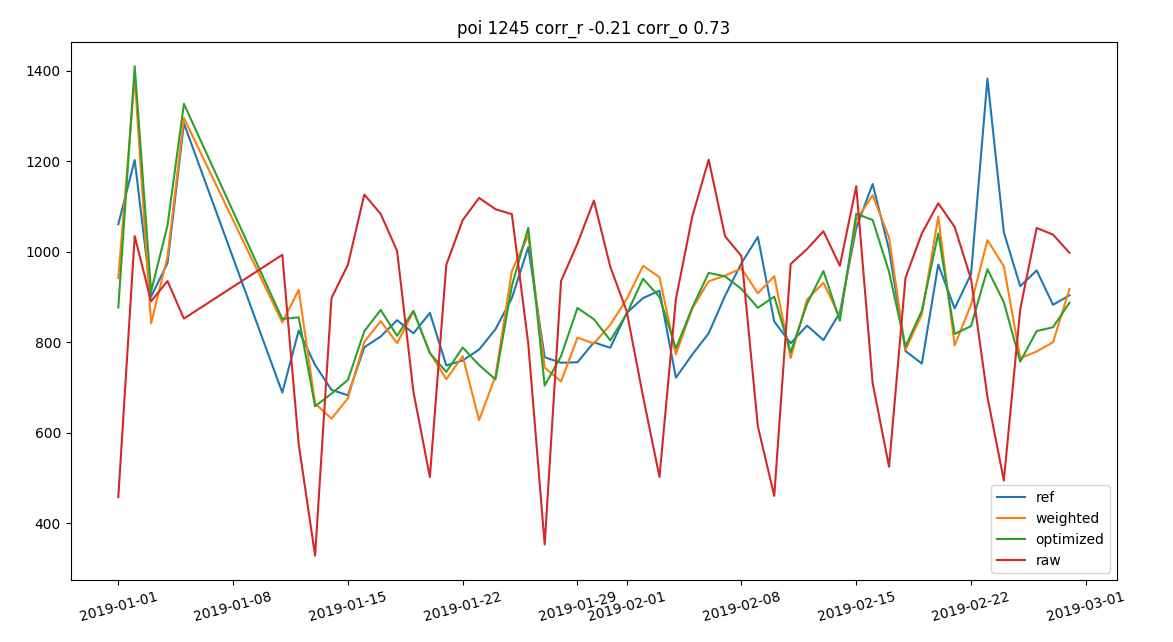linear regression on cells

## effect of filtering

We iterate over the different filters and we see a slight improvement in input data which is, on the other hand, irrelevant after weighting.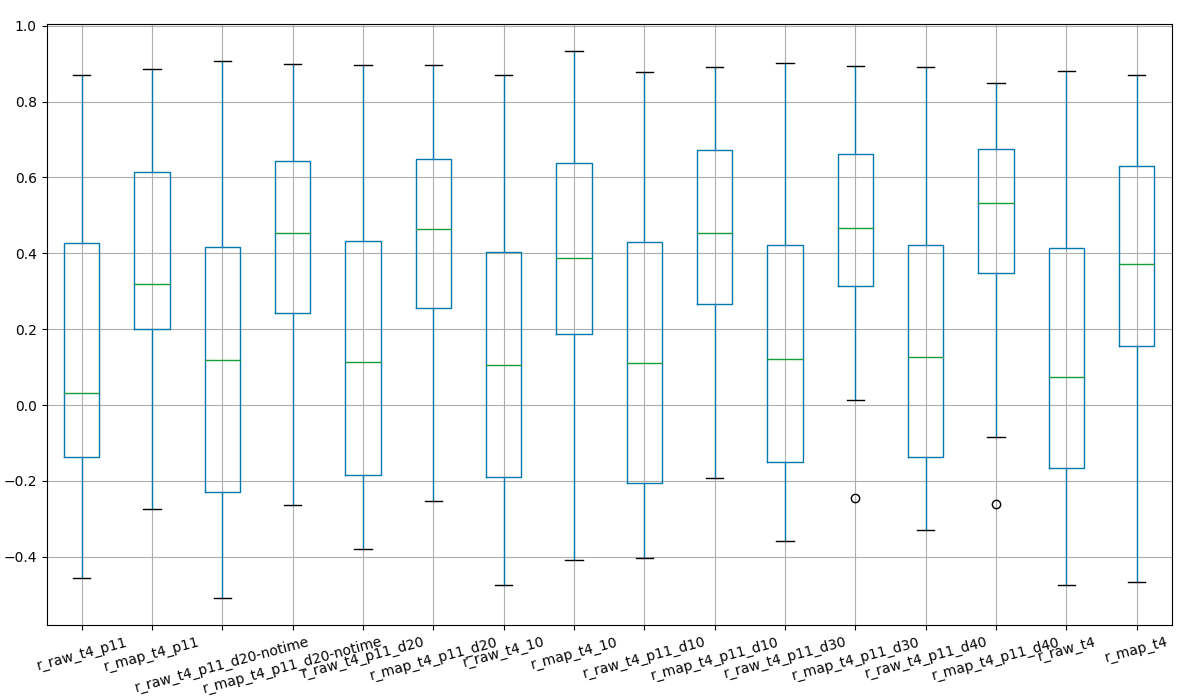effect of filtering on correlation pre and post mapping

Among all the different combination we prefere the version 11.6.1, 40 km previous distance, chirality, 0 to 2h dwelling time.

We see that chirality is stable but a third of counts doesn’t have a chirality assigned.daily values of chirality

## effect of mapping

We monitor the effect of the different steps on the total correlation with reference values.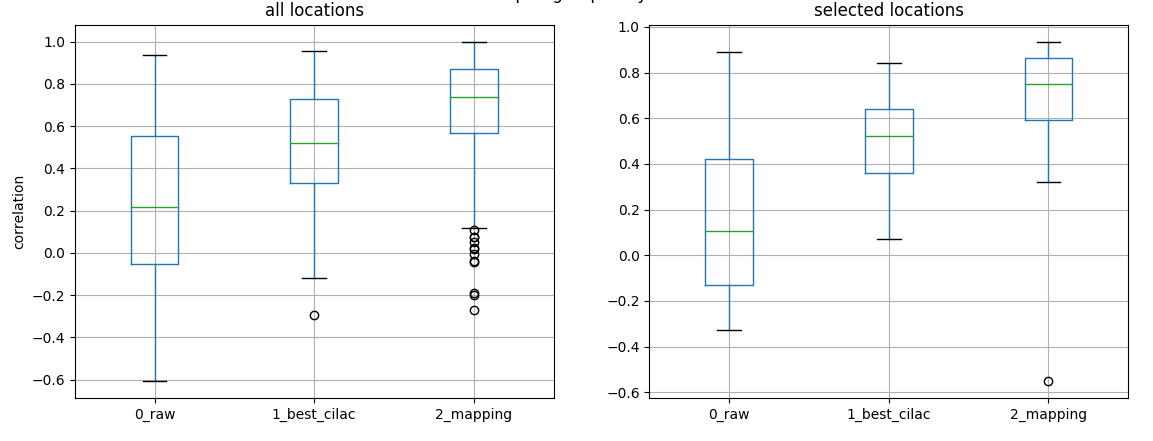correlation monitorin after any process step

## direction counts

We use etl_dirCount to download with beautifulSoup all the processd day from the analyzer output and create a query to the postgres database.

A spark function loads into all the database outputs into a pandas dataframe, take the maximum of the incoming flow and correct the timezone.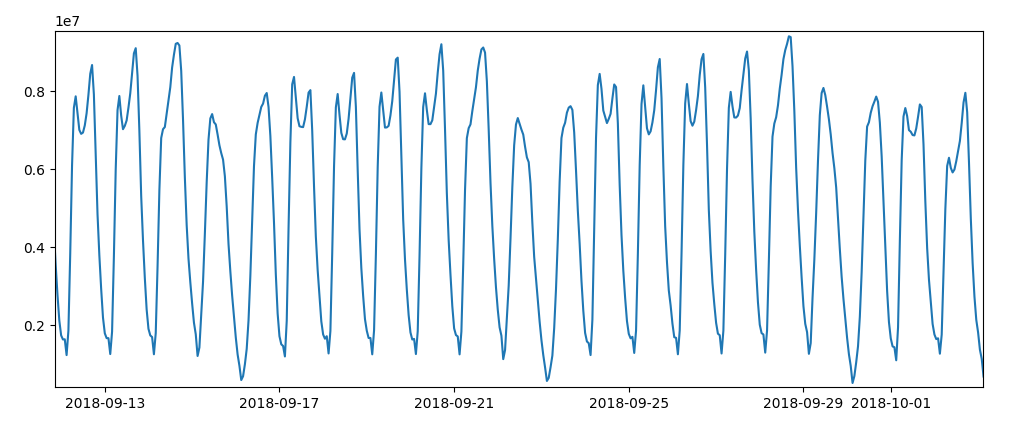direction counts

## capture rate

Now we check the stability of the capture rate over time and the min-max interval on monthly and daily values.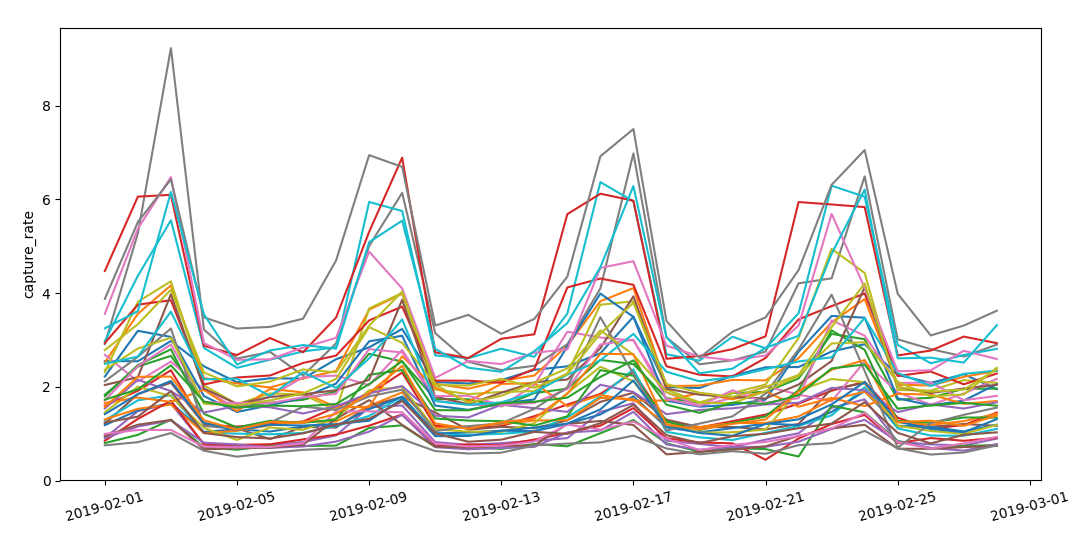capture rate over different locations which show a common pattern

We see that the range of maximum and minimum capture rate is similar over different locations and we don’t have problematic outliers.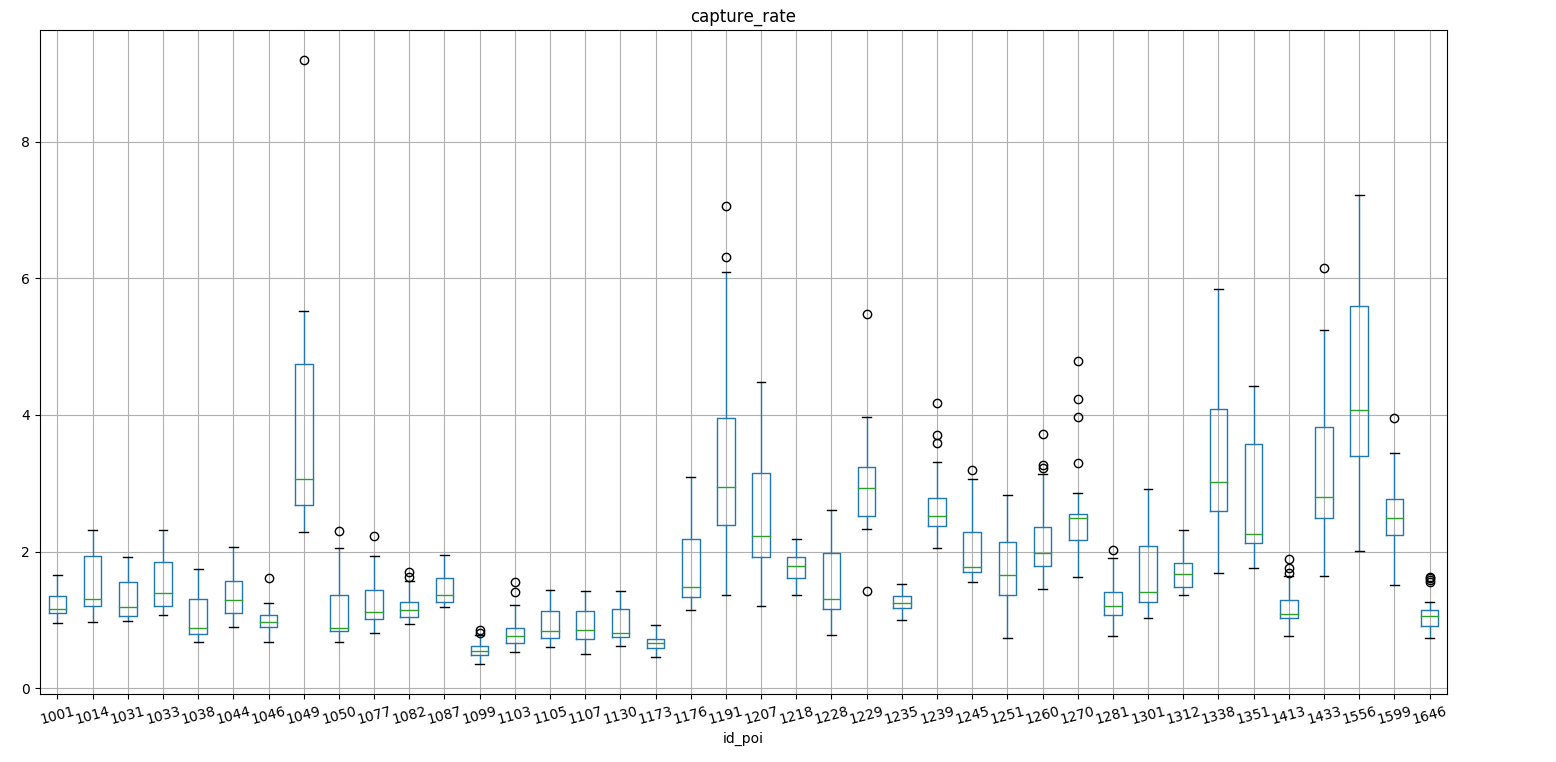capture rate boxplot over different locations

## Software

Over the time different models were tested to select the one with the best performances and stability.

The suite basically consists in:

## learn play

We use learn_play to run a series of regressor on the time series.

Depending on the temporal resolution we use a different series of train_execute

We use kpi_viz to visualize the performances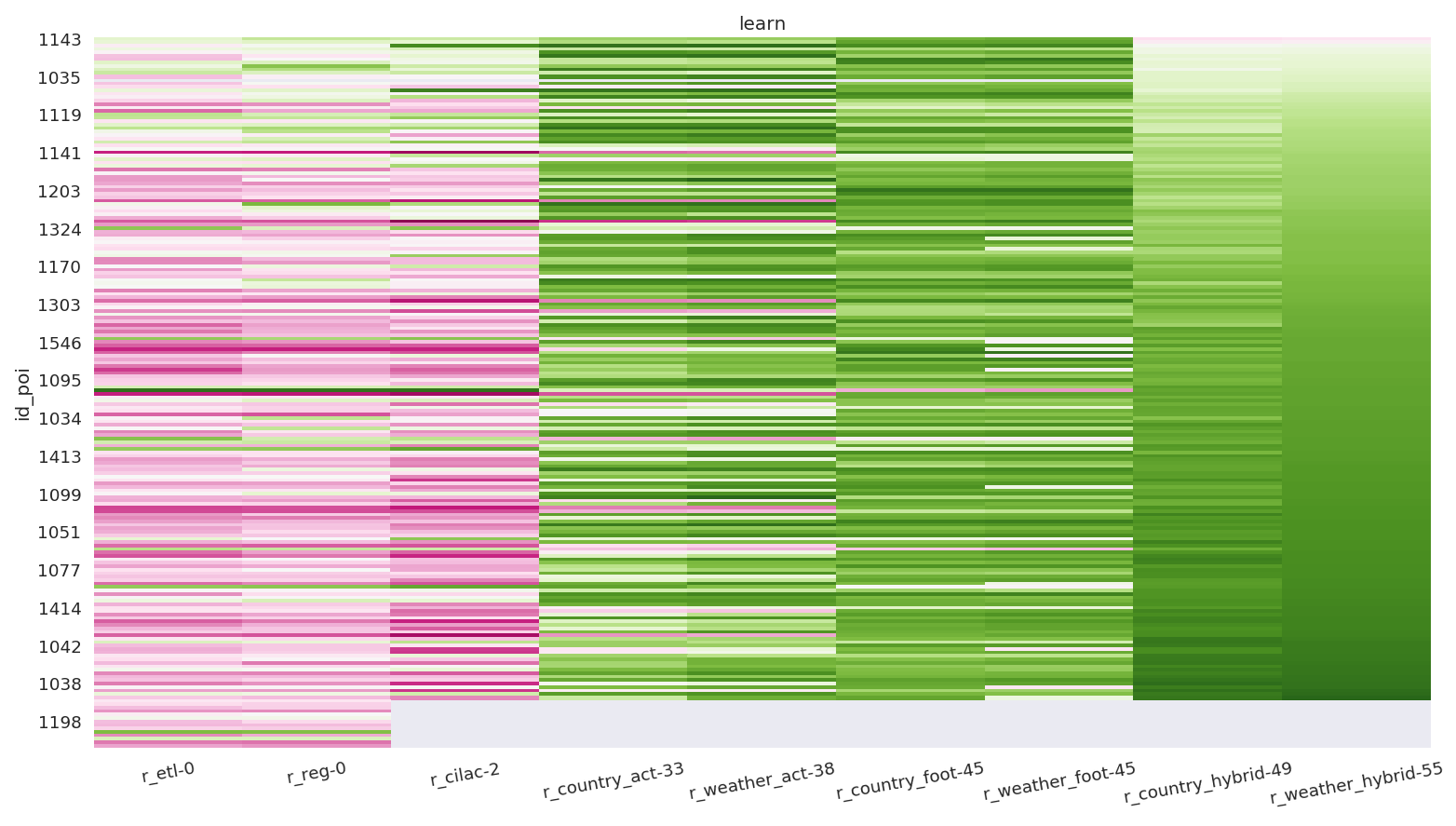kpi boost

## Long short term memory

We prepare a dataset overlapping the following information:

• reference data
• time
• activities
• footfall
• bast (isocalendar)
• historical data (isocalendar)
• mean temperature
• cloud cover
• humidity

We can see that not only all data sources are presents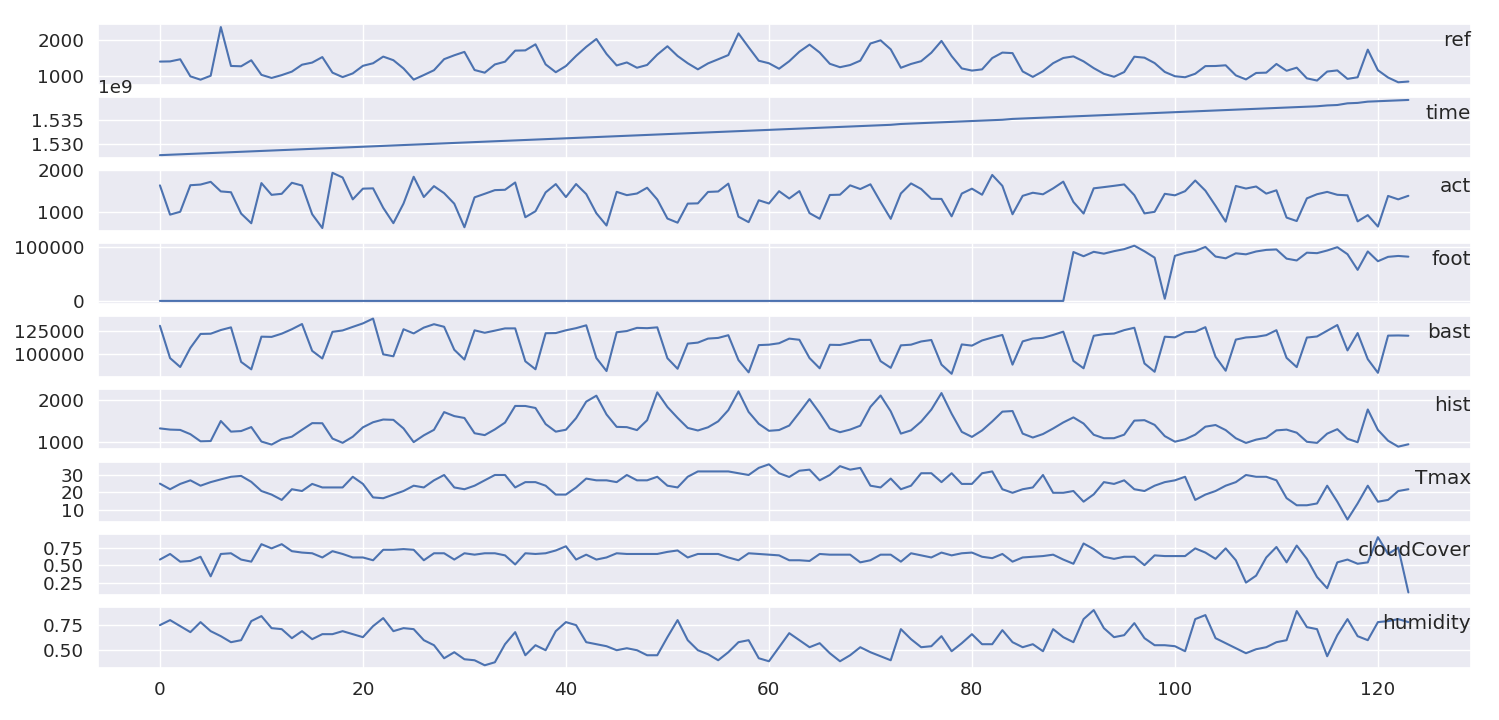input data

We than iterate over all locations and run a forecast over 30 days using a long short term memory algorithm train_longShort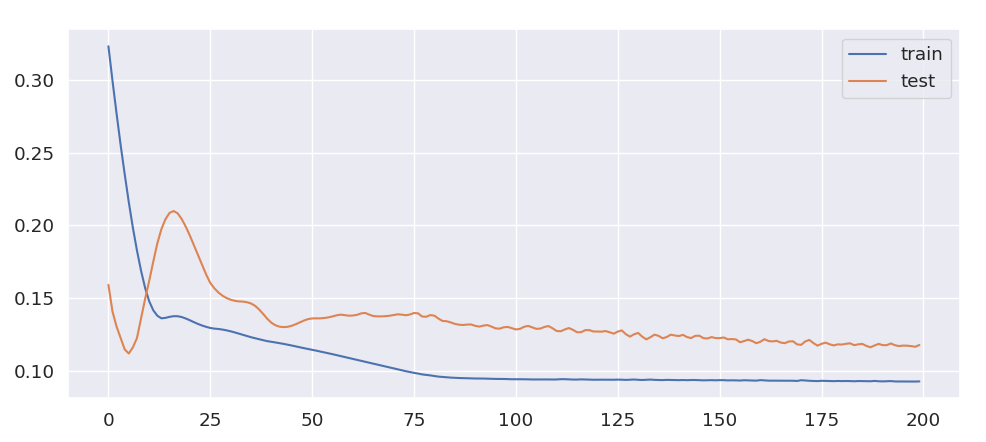performance history

We can see that the learning curve is pretty steep at the beginning and converges towards the plateau of the training set performance.

## First location set

We start preparing the data for a first set of location where:

• high number of daily visits
• low chi square, skewness, variance on reference
• high correlation blind test
• low variance long short term memory
• large cell coverage

We summarized the most important metrics in this graphrange of scores, relative values, 0 as minimum, areas represent confidence interval

and summarized in this table.

Performances depend on the regularity of input data over time.

## Production

The cooperation with insight is required for:

• backup in the project [short term]
• validity proof [short term]
• optimization of methods [short term]
• productization [long term]
• harmonization of the xy source [short term]
• impact of new infrastructure on activities and footfall [short term]
• improve spatial resolution for activities [long term]
• improve activity filters [long term]

TODOs:

• feature importance for every location
• evaluate the model on isocalendar for feb 2019
• retrain on new reference data and include footfall

## Competitors

After the finalization of the prediction of the reference data another prediction model has to be built to derive motorway guest counts for competitor locations.

This model will take into consideration:

• telco data
• cilac labelling
• geographical data (population density, land use)
• weather data